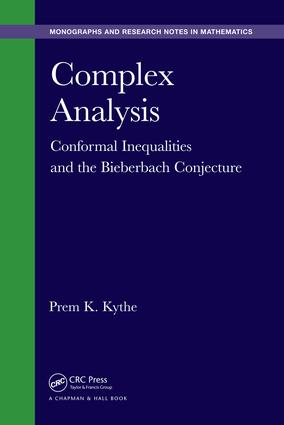# Complex Analysis

## Conformal Inequalities and the Bieberbach Conjecture, 1st Edition

Chapman and Hall/CRC

343 pages | 33 B/W Illus.

##### Purchasing Options:\$ = USD
Hardback: 9781498718974
pub: 2015-12-22
SAVE ~\$24.00
\$120.00
\$96.00
x
eBook (VitalSource) : 9780429171567
pub: 2016-04-19
from \$28.98

FREE Standard Shipping!

### Description

Complex Analysis: Conformal Inequalities and the Bieberbach Conjecture discusses the mathematical analysis created around the Bieberbach conjecture, which is responsible for the development of many beautiful aspects of complex analysis, especially in the geometric-function theory of univalent functions. Assuming basic knowledge of complex analysis and differential equations, the book is suitable for graduate students engaged in analytical research on the topics and researchers working on related areas of complex analysis in one or more complex variables.

The author first reviews the theory of analytic functions, univalent functions, and conformal mapping before covering various theorems related to the area principle and discussing Löwner theory. He then presents Schiffer’s variation method, the bounds for the fourth and higher-order coefficients, various subclasses of univalent functions, generalized convexity and the class of α-convex functions, and numerical estimates of the coefficient problem. The book goes on to summarize orthogonal polynomials, explore the de Branges theorem, and address current and emerging developments since the de Branges theorem.

### Table of Contents

Analytic Functions

Definitions

Jordan Contour

Cauchy–Riemann Equations

Line Integrals

Cauchy’s Theorems

Harmonic Functions

Piecewise Bounded Functions

Metric Spaces

Equicontinuity

Univalent Functions

Conformal Mapping

Some Theorems

Implications

Analytic Continuation

Schwarz Reflection Principle

Bilinear Transformation

Poisson’s Formula

Area Principle

Area Theorems

Bieberbach Conjecture

Lebedev–Milin’s Area Theorem

Koebe’s Theorem

Grunsky Inequalities

Polynomial Area Theorem

Distortion Theorems

Criterion for Univalency

Schwarzian Derivative

Löwner Theory

Carathéodory’s Kernel Theorem

Löwner’s Theorem

Löwner–Kufarev Equation

Applications

Slit Mappings

Higher-Order Coefficients

Variation Method

Fourth Coefficient

Grunsky Matrix

Higher-Order Coefficients

Subclasses of Univalent Functions

Basis Classes

Functions with Positive Real Part

Functions in Class S0

Typically Real Functions

Starlike Functions

Functions with Real Coefficients

Functions in Class Sa

Generalized Convexity

Convex Functions

Close-to-Convex Functions

γ-Spiral Functions

Generalized Convexity

Alpha-Convex Functions

Coefficients Estimates

Mean Modulus

Hayman Index

Conformal Inequalities

Exponentiation of Inequalities

Polynomials

Orthogonal Polynomials

Hypergeometric Functions

Faber Polynomials

De Branges Theorem

Conjectures

de Branges Theorem

Alternate Proofs of de Branges Theorem

de Branges and Weinstein Systems of Functions

Epilogue: After de Branges

Chordal Löwner Equations

Löwner Curves

Löwner Chains in Cn

Multivariate Holomorphic Mappings

Beurling Transforms

Appendix A: Mappings

Appendix B: Parametrized Curves

Appendix C: Green’s Theorems

Appendix D: Two-Dimensional Potential Flows

Appendix E: Subordination Principle

Exercises appear at the end of each chapter.

### About the Author

Prem K. Kythe is a Professor Emeritus of Mathematics at the University of New Orleans. He is the author/coauthor of 11 books and author of 46 research papers. His research interests encompass the fields of complex analysis, continuum mechanics, and wave theory, including boundary element methods, finite element methods, conformal mappings, PDEs and boundary value problems, linear integral equations, computation integration, fundamental solutions of differential operators, Green’s functions, and coding theory.

### Subject Categories

##### BISAC Subject Codes/Headings:
MAT037000
MATHEMATICS / Functional Analysis